Pure and Applied Mathematics Journal
Volume 4, Issue 3, June 2015, Pages: 75-79

Refined Definitions in Real Numbers and Vectors and Proof of Field Theories

Edward T. H. Wu

DaVinci International Academy, Los Angeles, USA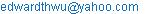Edward T. H. Wu. Refined Definitions in Real Numbers and Vectors and Proof of Field Theories. Pure and Applied Mathematics Journal. Vol. 4, No. 3, 2015, pp. 75-79. doi: 10.11648/j.pamj.20150403.13

Abstract: A set of new and refined principles and definitions in Real Numbers and Vectors are presented. What is a Vector? What is the meaning of the Addition of two Vectors? What is a Real Number? What is the meaning of their signs? What is the meaning of the Addition of two Real Numbers? What is the Summation Principle in Addition Operation? What is the Cancellation Principle in Addition Operation? What is the Meaning of the Multiplication of two Real Numbers? Is Field Theory a law? Can it be proved? All these issues are addressed in this paper. With better pictures and graphical presentations, proof of Field Theories in Real Numbers and Vectors including Commutativity, Associativity and Distributivity are also proposed.

Keywords: Vector, Real Number, Number Line, Number Vector, Number Plane, Number Space, Summation Principle, Cancellation Principle, Field Theory, Commutativity, Associativity, Distributivity

1. Definitions of Vectors

Definition 1: Vector X

Vector X (Ref. 1) (Ref. 2) (Ref. 3) is a straight arrow starting from the origin and ending at a point in the space which contains two components, direction and length (amount of Unit Length).

Definition 2: Addition of Vectors X + Y

X + Y is a Vector with its starting point at the origin and the ending point that follows X continuously to the end of Y’, where Y’ is a parallel Vector of Y (Fig. 1).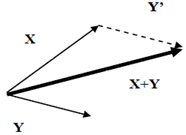Fig. 1. Definition of X + Y.

Definition 3: Negative Vector -(X)

-(X) + X = 0

-(X) is a Vector that the addition of -(X) and X is 0 (0 Vector is a Vector starting from origin and ending at origin) (Fig. 2).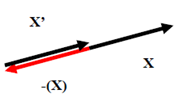Fig. 2. Definition of –(X).

Definition 4: Multiplication of Vector aX

If a > 0, then aX is a Vector that starts at origin and has a length equals to the amount /a/ (absolute value) times /X/ (length of X) with the same direction of X.

If a < 0, then aX is a Vector that has a length equals to the amount /a/ times /X/ (length of X) with an opposite direction of X (Fig.3).Fig. 3. Definition of aX.

[Theory of Vectors]

-aX = -(aX)

Because -aX + aX = 0 and –(aX) + aX = 0

Therefore, -aX = -(aX)

Also, -X = -(X)

Where -X = -1X

2. Definitions of Real Numbers

Definition 1. Real Numbers

A Real Number (Ref 4) (Ref. 5) (Ref. 6) "a" contains two components, the amount of Unit Element (/a/) and the sign of Unit Element (Positive or Negative). For example, "+5" represents "5" pieces of "Positive" Unit Element (where 5 = /+5/ is the amount and Positive "+" is the sign), and "-5" represents "5" pieces of "Negative" Unit Element (where 5 = /-5/ is the amount and Negative "-" is the sign).

2.1. Number Line

Number Line (Ref. 7) (Ref. 8) is a line of Real Numbers on one dimensional Cartesian coordination system with positive numbers on the right hand side and negative numbers on the left hand side of the origin. For any Real Number "a", there is a coordinating "Point a" and "Line a" between origin and "Point a" on the Number Line, where the amount of the "Unit Length" contained in "Line a" equals to the amount /a/. The sign of the "Unit Length" is positive for those on the right hand side and negative for those on the left hand side of the origin, which is the same as that of "a".

2.2. Number Vector

For any Real Number "a", there is a Number Vector "aU" on the Number Line with origin as its starting point and the coordinating "Point a" as its ending point. The Number Vector of positive "a" has direction towards right and the Number Vector of negative "a" has direction towards left of the origin. Length /aU/ the amount of the "Unit Length" in "aU", equals to /a/ the amount of "Unit Element" in "a". The direction of "aU" coincides with the sign of  "Unit Length" in "aU" and also the sign of  "Unit Element" in "a".

2.3. Number Plane

Number Plane is a plane of Real Numbers on two dimensional Cartesian coordinate system. For any point (a, b) in the Number Plane, there is a corresponding Real Number "m" which has amount /m/ equals to the amount /a//b/ of the "Unit Square" [1x1] contained in the rectangular [a x b]. The sign of the "Unit Square" is the same as that of "m" defined by its position (a, b) in Fig. 5.

For any Real Number "a", there is a coordinating point (a, 1) and rectangular [a x 1] in the X axis Number Plane [X x1], which has the amount of "Unit Square" [1x1] in [a x 1] equals to /a/. The sign of the "Unit Square" is the same as that of "a" and (a, 1) in Fig. 5.

2.4. Number Space

Number Space is a space of Real Numbers on three dimensional Cartesian coordinate system. For any point (a, b, c) in the Number Space, there is a corresponding Real Number "m" which has amount /m/ equals to the amount /a//b//c/ of the "Unit Cube" [1x1x1] contained in the orthorhombic [a x b x c]. The sign of the "Unit Cubic" is the same as that of "m" defined by its position (a, b, c) in Fig. 5.

For any number "a", there is a coordinating point (a, 1, 1) and orthorhombic [a x 1 x 1] in the X axis Number Space [X x1x1], which has the amount of "Unit Cube" [1x1x1] in [a x 1 x 1] equals to /a/. The sign of the "Unit Cubic" is the same as that of "a" and (a, 1, 1) in Fig. 5.

In summary, for any Real Number "a", there are corresponding domains "Line a", "aU", "[a x 1]" and "[a x 1 x 1]" in Number Line, Number Vector, Number Plane and Number Space respectively that the amount of the "Unit Element" equals to the amount of "Unit Length", "Unit Square" and "Unit Cube". Also, the sign of the "Unit Element" is the same as that of the "Unit Length", "aU", "Unit Square" and "Unit Cube".

In Addition Operation, for any two Real Numbers of the same signs, all Unit Elements coexists and contributes to the final total amount.  This is called "Summation Principle". In Number Vectors, the Summation Principle of the Real Numbers is processed automatically through the operation of Addition of Vectors.

In Addition Operation, for any two Real Numbers of opposite signs, each Positive Unit Element cancels out one Negative Unit Element to achieve the net amount of the total Unit Elements. This is called "Cancellation Principle". In Number Vectors, the Cancellation Principle of the Real Numbers is also processed automatically through the operation of Addition of Vectors.

Definition 2. Addition a + b

In Addition Operation of two Real Numbers, for "a" and "b" of the same signs, according to the Summation Principle, "a + b" represents a Real Number with the amount of its "Unit Element" equals to the total amount (/a/ + /b/) of the "Unit Element" in "a" and "b", and a sign of its "Unit Element" the same as that of "a" and "b". For "a" and "b" of the opposite signs, according to the Cancellation Principle, "a + b" represents a Real Number with the amount of its "Unit Element" equals to the difference between the two amounts (/(/a/-/b/)/) of  the "Unit Element" in "a" and "b", and a sign of its "Unit Element" is the same as that of the larger amount. For examples, (+7) + (+6) = (+13), (-2) + (-5) = (-7), (+7) + (-6) = (+1) and (+5) + (-8) = (-3).

More specifically,

If a>0 & b>0

a + b >0 & /a + b/ = /a/ + /b/;

If a<0 & b<0

a + b <0 & /a + b/ = /a/ + /b/;

If a>0 & b<0 & /a/ > /b/

a + b >0 & /a + b/ = /a/ - /b/;

If a>0 & b<0 & /a/ < /b/

a + b <0 & /a + b/ = /b/ - /a/;

If a<0 & b>0 & /a/ > /b/

a + b <0 & /a + b/ = /a/ - /b/;

If a<0 & b>0 & /a/ < /b/

a + b >0 & /a + b/ = /b/ - /a/;

[Theory of Vectors]

aU + bU = (a + b)U

For "a" and "b" of the same signs, according to the definition of the Addition of Vectors, "aU + bU" represents a Number Vector with the amount of its "Unit Length" equals to the total amount (/a/ + /b/) of the "Unit Length" in "aU" and "bU", and a sign of its "Unit Length" the same as that of "a" and "b". Similarly, "(a + b)U" represents a Number Vector with the amount of its "Unit Length" equals to the amount of the "Unit Element" in "(a + b)" which is the total amount (/a/ + /b/) of the "Unit Element" in "a" and "b", and a sign of its "Unit Length" the same as that of "(a + b)" also as that of  "a" and "b".

For "a" and "b" of the opposite signs, according to the definition of the Addition of Vectors, "aU + bU" represents a Number Vector with the amount of its "Unit Length" equals to the difference between the two amounts (/(/a/-/b/)/) of the "Unit Length" in "aU" and "bU", and a sign of its "Unit Length" the same as that of the larger amount. Similarly, "(a + b)U" represents a Number Vector with the amount of its "Unit Length" equals to the difference between the two amounts (/(/a/-/b/)/) of the "Unit Element" in "a" and "b", and a sign of its "Unit Length" the same as that of the larger amount.

In both cases, the amount and sign of the "Unit Length" in aU + bU are the same as that of (a + b)U, therefore aU + bU = (a + b)U (Fig. 4).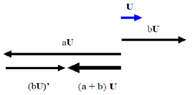Fig. 4. aU + bU = (a + b)U.

Definition 3. Negative -(a)

-(a) + a = 0

[Theory of Vectors]

-(a)U + aU = 0U

Because -(a)U + aU = (-(a) + a)U = 0U

[Theory of Real Numbers]

-a = -(a)

Because -aU + aU = 0U

and -(a)U + aU = 0U

Therefore, -a = -(a)

Definition 4. Multiplication a x b

"a x b" is a Real Number in Number Plane with its amount /a x b/ equals to /a/ x /b/, represents the total amount of Unit Squares [1 x 1] in the rectangular [a x b] along X and Y axes. Also in Number Space, it represents the total amount of Unit Cubes [1 x 1 x 1] in the orthorhombic [a x b x 1] along X, Y and Z axes. The sign of "a x b" is defined by the following method also as that in Number Space (Fig. 5).

a    b      a x b

(+) (+) = (+)

(+) (−) = (−)

(−) (+) = (−)

(−) (−) = (+)

[Multiplication (a x b) x c]

According to the definition of Multiplication (a x b), it implies that (a x b) x c is also a Real Number in Number Space with its amount /(a x b) x c/ equals to (/a/ x /b/) x /c/, represents the total amount of Unit Cubes in the orthorhombic [a x b x c] along X, Y and Z axes. The sign of "(a x b) x c" is determined by the following method also as that in Number Space (Fig. 5).

a    b    c       (a x b) x c

(+) (+) (+) = (+)

(+) (−) (+) = (−)

(−) (+) (+) = (−)

(−) (−) (+) = (+)

(+) (+) (−) = (−)

(+) (−) (−) = (+)

(−) (+) (−) = (+)

(−) (−) (−) = (−)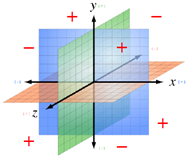Fig. 5. Signs of (a x b x c).

3. Field Theories of Real Numbers

In Real Numbers, with the definitions of Addition and Multiplication, Field Theories (Ref. 9) (Ref. 10) can be proved as follows:

3.1. Commutativity of Addition a + b = b + a

Beause

aU + bU = (a+b)U

bU + aU = (b+a)U

also, X + Y = Y + X (Fig. 7),

Therefore, a+b = b+a

3.2. Commutativity of Multiplication a x b = b x a

Because both the amount and sign of the Unit Squares in rectangular (/a/ x /b/) and (/b/ x /a/) are the same,

If a>0 & b>0

a x b = /a/ x /b/

b x a = /b/ x /a/

Therefore, a x b = b x a;

If a>0 & b<0

a x b = /a/ x -/b/ = - (/a/ x /b/)

b x a = -/b/ x /a/ = - (/b/ x /a/)

Therefore, a x b = b x a;

If a<0 & b>0

a x b = -/a/ x /b/ = - (/a/ x /b/)

b x a = /b/ x -/a/ = - (/b/ x /a/)

Therefore, a x b = b x a;

If a<0 & b<0

a x b = -/a/ x -/b/ = /a/ x /b/

b x a = -/b/ x -/a/ = /b/ x /a/

Therefore, a x b = b x a;

3.3. Associativity of Addition (a + b) + c = a + (b + c)

((a + b) + c)U = (a + b)U + cU = (aU + bU) +cU

(a + (b + c))U = aU + (b + c)U = aU + (bU + cU)

Because (X + Y) + Z = X + (Y + Z) (Fig. 8)

((a + b) + c)U = (a + (b + c))U

Therefore, (a + b) + c = a + (b + c)

3.4. Associativity of Multiplication (a x b) x c = a x (b x c)

Because both the amount and sign of the Unit Cubes in orthorhombic ((/a/ x /b/) x /c/) and (/a/ x (/b/ x /c/)) are the same,

For c > 0,

If a>0 & b>0

(a x b) x c = (/a/ x /b/) x /c/

a x (b x c) = /a/ x (/b/ x /c/)

Therefore, (a x b) x c = a x (b x c);

If a>0 & b<0

(a x b) x c = (/a/ x -/b/) x /c/ = - ((/a/ x /b/) x /c/)

a x (b x c) = /a/ x (-/b/ x /c/) = - (/a/ x (/b/ x /c/))

Therefore, (a x b) x c = a x (b x c);

If a<0 & b>0

(a x b) x c = (-/a/ x /b/) x /c/ = - ((/a/ x /b/) x /c/)

a x (b x c) = -/a/ x (/b/ x /c/) = - (/a/ x (/b/ x /c/))

Therefore, (a x b) x c = a x (b x c);

If a<0 & b<0

(a x b) x c = (-/a/ x -/b/) x /c/ = (/a/ x /b/) x /c/

a x (b x c) = -/a/ x (-/b/ x /c/) = (/a/ x (/b/ x /c/)

Therefore, (a x b) x c = a x (b x c);

For c <0,

If a>0 & b>0

(a x b) x c = (/a/ x /b/) x -/c/ = - ((/a/ x /b/) x /c/)

a x (b x c) = /a/ x (/b/ x -/c/) = - (/a/ x (/b/ x /c/))

Therefore, (a x b) x c = a x (b x c);

If a>0 & b<0

(a x b) x c = (/a/ x -/b/) x -/c/ = (/a/ x /b/) x /c/

a x (b x c) = /a/ x (-/b/ x -/c/) = /a/ x (/b/ x /c/)

Therefore, (a x b) x c = a x (b x c);

If a<0 & b>0

(a x b) x c = (-/a/ x /b/) x -/c/ = (/a/ x /b/) x /c/

a x (b x c) = -/a/ x (/b/ x -/c/) = /a/ x (/b/ x /c/)

Therefore, (a x b) x c = a x (b x c);

If a<0 & b<0

(a x b) x c = (-/a/ x -/b/) x -/c/ = - ((/a/ x /b/) x /c/)

a x (b x c) = -/a/ x (-/b/ x -/c/) = - (/a/ x (/b/ x /c/))

Therefore, (a x b) x c = a x (b x c);

3.5. Distributivity (a + b) x c = a x c + b x c

For two Real Numbers "a" and "b" of the same signs, "a x c", "b x c" and "(a + b) x c" also have the same signs. According to the "Summation Principle", both "a x c + b x c" and "(a + b) x c" represents the total amount of the "Unit Squares" of the same sign.

However, for "a" and "b" of the opposite signs, "a x c" and "b x c" also have the opposite signs. According to the "Cancellation Principle", both "a x c + b x c" and "(a + b) x c" represents the difference between the two amounts of opposite Unit Squares with a sign follows that of the larger amount between "a" and "b".

As shown in Fig. 6, (a + b)U = aU + bU, where aU is the blue Vector, bU is the red Vector and  (a + b)U is the green Vector. Also, "a x c" represents the amount of Unit Squares in Blue rectangular area upon "a" and "b x c" represents the amount of Unit Squares in Red rectangular area upon "b". In Addition Operation, because "(a + b) x c" represents the amount of Unit Squares in Green rectangular area upon "a + b". Also, "a x c + b x c" represents the same amount of Unit Squares in Green rectangular area resulting from the Addition Operation of "a x c" and "b x c", therefore, (a + b) x c = a x c + b x c.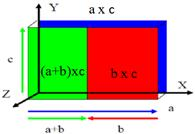Fig. 6. Proof of (a + b) x c = a x c + b x c.

4. Field Theory of Vectors

In Vectors, with the definitions of Vectors and Addition, Field Theories of Vectors (Ref. 11) (Ref. 12) (Ref. 13) can be proved as follows:

4.1. Commutativity  X + Y = Y + X

Because X//X’ & Y//Y’ (Fig. 7)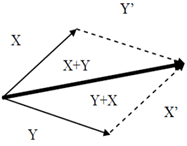Fig. 7. Proof of X + Y = Y + X.

4.2. Associativity (X + Y) + Z = X + (Y + Z)

Because (Y+Z)’= Y’ + Z’ (Fig. 8)Fig. 8. Proof of (X + Y) + Z = X + (Y + Z).

4.3. Distributivity

(a + b)X = aX + bX

a(X + Y) = aX + aY

Because Y’ // aY’ (Fig. 9)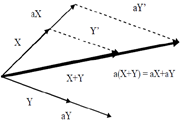Fig. 9. Proof of a(X + Y) = aX + aY.

5. Other Theories of Real Numbers

The following theories can be easily proved by definitions and graphic presentations.

a + 0 = a

- (-(a)) = a

- (a+b) = (-a) + (-b)

1a = a

a a-1= 1

(a-1)-1 = a

(ab)-1=a-1b-1

0 a = 0

6. Other Theories of Vectors

The following theories can be easily proved by definitions and graphic presentations.

0X = 0

X + 0 = X

-(-X) = X

-(X+Y) = (-X) + (-Y)

References

1. Galbis, Antonio & Maestre, Manuel (2012). Vector Analysis Versus Vector Calculus. Springer. p. 12. ISBN 978-1-4614-2199-3.
2. J.E. Marsden (1976). Vector Calculus. W. H. Freeman & Company. ISBN 0-7167-0462-5.
3. http://en.wikipedia.org/wiki/Vector_calculus.
4. Solomon Feferman, 1989, The Numbers Systems: Foundations of Algebra and Analysis, AMS Chelsea, ISBN 0-8218-2915-7.
5. Howie, John M., Real Analysis, Springer, 2005, ISBN 1-85233-314-6.
6. http://en.wikipedia.org/wiki/Real_number.
7.  Stewart, James B.; Redlin, Lothar; Watson, Saleem (2008). College Algebra (5th ed.). Brooks Cole. pp. 13–19. ISBN 0-495-56521-0.
8. http://en.wikipedia.org/wiki/Number_line.
9. http://en.wikipedia.org/wiki/Field_(mathematics)
10. Jacobson, Nathan (2009), Basic algebra 1 (2nd ed.), Dover, ISBN 978-0-486-47189-1.
11. http://en.wikipedia.org/wiki/Linear_algebra
12. http://en.wikipedia.org/wiki/Vector_space
13. Lang, Serge (1987), Linear algebra, Berlin, New York: Springer-Verlag, ISBN 978-0-387-96412-6.

 Contents 1. 2. 2.1. 2.2. 2.3. 2.4. 2.5. 2.6. 3. 3.1. 3.2. 3.3. 3.4. 3.5. 4. 4.1. 4.2. 4.3. 5. 6.
Article ToolsAbstractPDF(458K)